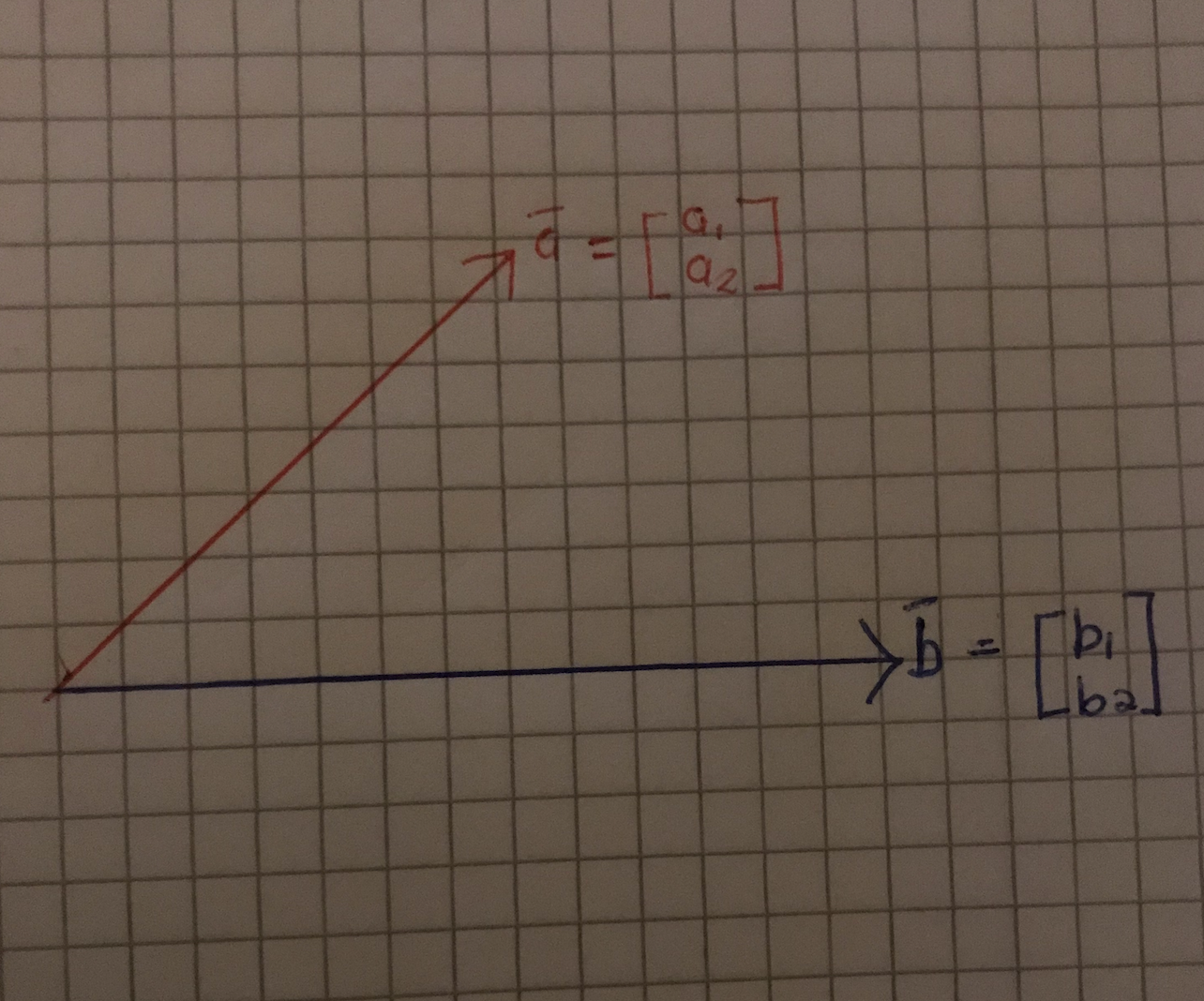# Support Vector Machines — Lecture series — Dot product cont’d

In the previous post we spoke about the concept of the dot product and what it means. In this post, we would be looking at how to actually calculate the dot product of two vectors.

Learning objective:

Know the 2 different ways in which the dot product can be expressed and understand how to obtain the dot product of two vectors algebraically

Main question:

Consider the 2 vectors in Fig 1 below, what are the ways in which you could actually obtain a value to represent the dot product of these 2 vectors?Fig 1

There are 2 ways in which you could obtain the dot product of these 2 vectors. You could decide to use the algebraic approach or the geometric approach.

In this post, we would be talking about how to use the algebraic approach. In this approach, the dot product of the vectors a and b can be calculated as:

a1b1 + a2b2

A more general formula for obtaining the dot product of 2 vectors (A and B)algebraically is:

In the next post, we will be talking about how to use the geometric approach to compute for the dot product between two vectors.